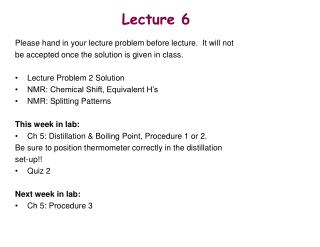DownloadDownload PresentationLecture 6

# Lecture 6

Download Presentation## Lecture 6

- - - - - - - - - - - - - - - - - - - - - - - - - - - E N D - - - - - - - - - - - - - - - - - - - - - - - - - - -
##### Presentation Transcript

1. Lecture 6 Please hand in your lecture problem before lecture. It will not be accepted once the solution is given in class. • Lecture Problem 2 Solution • NMR: Chemical Shift, Equivalent H’s • NMR: Splitting Patterns This week in lab: • Ch 5: Distillation & Boiling Point, Procedure 1 or 2. Be sure to position thermometer correctly in the distillation set-up!! • Quiz 2 Next week in lab: • Ch 5: Procedure 3

2. Nuclear Magnetic Resonance Information Gained: • Different chemical environments of nuclei being analyzed (1H nuclei): chemical shift • The number of nuclei with different chemical environments: numberof signals in spectrum • The numbers of protons with the same chemical environment: integration • Determine how many protons are bonded to the same carbon: integration • Determine the number of protons that are adjacent to one another: splitting patterns • Determine which protons are adjacent to one another: coupling constants

3. Chemical Shifts • Shielded protons appear more upfield (lower ppm value). • Deshielded protons appear more downfield (higher ppm value). Correlation Chart

4. Chemical Shifts TMS - Tetramethylsilane (Me4Si) is the internal reference used in many examples. TMS’s chemical shift is set at zero since most peaks appear more downfield from it. (Note: You will use your NMR solvent, CDCl3, as your reference peak. Why use CDCl3 to make up your sample for NMR?) • The Delta (d) Scale • An arbitrary scale • 1 d = 1 part per million (ppm) of the spectrometer operating frequency. • For example, if using an 80 MHz instrument to run a 1H NMR spectrum, • 1 d would be 1 ppm of 80,000,000 Hz, or 80 MHz. • Since the radiofrequency absorption of a nuclei depends on the magnetic • field strength, chemical shift in Hz would vary from instrument to • instrument. The stronger the field, the greater the DE (magnetic transition). • Thus, report the nuclei absorption in relative terms (d) as opposed to • absolute terms (Hz). This way, the chemical shifts will be the same • for nuclei of a sample despite what instrument you use - leads to • correlation charts!

5. 1H NMR Spectrum of Ethanol b a c a CH3CH2OH TMS Three signals - three different types of H’s b c downfield upfield ppm

6. Equivalent & Non-Equivalent Hydrogens As seen in the 1H NMR spectrum of ethanol, the number of signals equals the number of different types of protons in a compound. • General rules: • Protons attached to the same sp3 carbon are equivalent (if there are no chiral • centers in the molecule; if there are, could be equivalent or non-equivalent). • If there is symmetry in the molecule, protons that are symmetrical will • have the same signal, the same chemical shift. • Note: Protons attached to the same sp2 carbon (in alkenes) need to be evaluated • for equivalency.

7. Equivalent & Non-Equivalent Hydrogens Consider the following molecules. Determine which protons are equivalent and non-equivalent. Predict the number of signals that would appear in the 1H NMR spectra of these compounds.

8. 1H NMR Spectrum of Ethanol: Spin-Spin Splitting b a c a CH3CH2OH TMS a - triplet b - quartet c - singlet b c downfield upfield ppm

9. Spin-Spin Splitting b a c • General rules: • Neighboring, non-equivalent protons split each • other’s signals • Equivalent protons do not split each other’s • signals • Use the n + 1 rule to predict the splitting • pattern of a proton’s signal CH3CH2OH a - triplet b - quartet c - singlet n + 1 rule The signal of a proton with n equivalent neighboring protons is split into a multiplet of n + 1 peaks. In ethanol, a neighbors b; they split each other’s peaks. Note that b neighbors c and no splitting occurs between the two; b is only affected by a. In general, protons that reside on heteroatoms (O, N) do not get involved with spin-spin splitting with neighboring protons. Thus, c appears as a singlet.

10. Spin-Spin Splitting Determine the splitting patterns for the signals in the 1H NMR spectra of the following compounds.